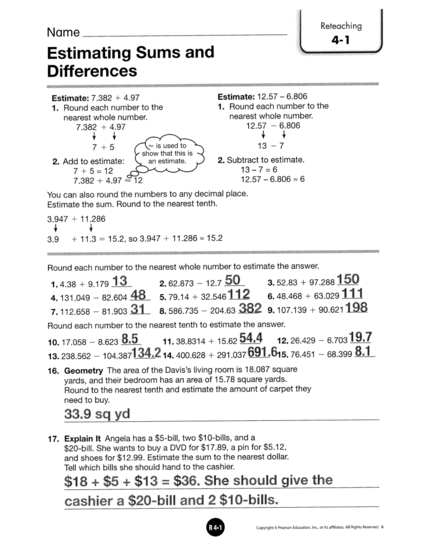# Estimating Sum and Difference of Decimal NumbersContributed by:Evaluating addition and subtraction expressions. The easiest way to estimate a sum or a difference of decimals is to round the decimal. You can do that by rounding each decimal to the nearest whole number. In 15.7, the place being rounded is the 5. Look at the 7 and round up.
1.
2.
3.
4.
5.
6.
7.
8.
9.
10.
11.
12.
13.
14.
15.
16.
17.
18.